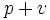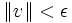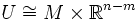Tubular neighborhood theorem

Let$M$ be a submanifold (differential sense) of$\R^n$, of dimension$m$. Then, there exists$\epsilon>0$ such that for any point at distance at most$\epsilon$ from$M$, there is a unique expression of the point as a sum$p + v$ where$p \in M$ and$v$ is a normal at$p$, with$\| v \| < \epsilon$.
If we define$U$ as the open subset of$\R^n$ comprising those points of$\R^n$ at distance less than$\epsilon$ from$M$, then$U$ can be viewed as a concrete realization, in the ambient space$\R^n$, of the normal bundle to$M$ in$\R^n$. In the situations where the normal bundle to$M$ is trivial, we see that this gives a natural diffeomorphism$U \cong M \times \R^{n-m}$.